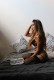703 209 9091

# How to make a form to calculate payments with Html and Java Script

### HTML/JavaScript form to calculate payments using APR

How much would you like to pay?:

 Negotiated price:* Sales tax:* % Term: 36 months48 months60 months Interest Rate:* % Down payment:

0

The form you see above calculates monthly payments, for example: car loan payments, etc.
It could be also used as a mortgage calculator if you make some changes to the formula.
Below you will find the HTML and Java Script code used to build the form.

### Html source code:

<form>
<td width=”94″>Negotiated price:*</td>
<td width=”165″><input type= “text” name=”nprice” /></td>
</tr>
<tr>
<td>Sales tax:*</td>
<td><input type=”text” name=”tax”/></td>
<td width=”53″>%</td>
</tr>
<tr>
<td height=”31″>Term:</td>
<td><select name=”term”>
<option value=”36″>36 months</option>
<option value=”48″>48 months</option>
<option value=”60″>60 months</option>
</select> </td>
</tr>
<tr>
<td>Interest Rate:*</td>
<td><label>
<input type=”irate” name=”rate”/>
</label> </td>
<td>%</td>
</tr>
<tr>
<td>Down payment:</td>
<td><label>
<input type=”downpayment” name=”payment” />
</label> </td>
</tr>
<tr>
<td>&nbsp;</td>
<td><input name=”Input” type=”button” value=”Calculate payments” onclick=”calcpayments()”/></td>
<td><input name=”reset2″ type=”reset” value=”Clear” /></td>
</tr>
</table>
<br />
<div id=”monthlypayment”></div>
</form>

### Java Script source code for the calculation:

<script>function calcpayments()

{

//The five variables we are going to use in the formula

var nprice=document.forms.nprice.value*1;
var salestax=document.forms.tax.value*1;
var interest=document.forms.rate.value*1;
var dpayment=document.forms.payment.value*1;

var t;

//We used a loop to select the term for the payments

for (i=0; i<document.forms.term.options.length; i++)

{

if (document.forms.term.options[i].selected)
t = document.forms.term.options[i].value*1;
}

//This is the formula that does the math

var result=(nprice*(salestax/100 +1)-dpayment)*((interest/100)/12) / (1-Math.pow((1+(interest/100)/12),(-t)));

result=Math.round(result*100) /100;

//The line below shows the result in the box

document.getElementById(“monthlypayment”).innerHTML=result;

}

</script>

You can try changing the term into years instead of months to make it work
for a home mortgage payment calculation.

1.2.